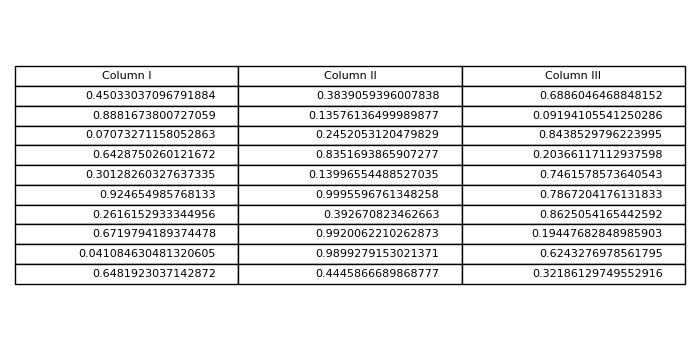# How do I plot only a table in Matplotlib?

To plot only a table, we can take the following steps−

• Create fig and axs, using subplots. Create a figure and a set of subplots.
• Create random data for 10 rows and 3 columns.
• Create a tuple for columns name.
• axis('tight') − Set the limits, just large enough to show all the data, then disable further autoscaling.
• axis('off') − Turn off axis lines and labels. Same as ''False''.
• To add a table on the axis, use table() instance, with column text, column labels, columns, and location=center.
• To display the figure, use show() method.

## Example

import numpy as np
from matplotlib import pyplot as plt
plt.rcParams["figure.figsize"] = [7.00, 3.50]
plt.rcParams["figure.autolayout"] = True
fig, axs = plt.subplots(1, 1)
data = np.random.random((10, 3))
columns = ("Column I", "Column II", "Column III")
axs.axis('tight')
axs.axis('off')
the_table = axs.table(cellText=data, colLabels=columns, loc='center')
plt.show()

## Output